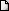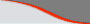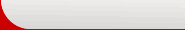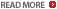CAT 2016 sample practice question bank Permutation, combination : Ascent Education CAT XAT course materialLATEST NEWS • Ascent Success Stories • TANCET 16 Classes @ Chennai • XAT 16 Classes @ ChennaiHome | TANCET Coaching Class | TANCET study material | Online TANCET Course | Contact us
 Ascent's Courses CAT 2010 for IIMs CAT Classroom Chennai Math & DI Crash Course CAT - Correspondence CAT Correspondence India CAT Corres Abroad Mock CAT Series CAT Crash Course Math Shortcut Workshops XAT Classes TANCET 2010 Classes MAT Classes GRE-GMAT GMAT Classes US B Schools Faculty List Question A Day Business Partnership Jobs @ Ascent Testimonials Ascent TANCET ToppersPermutation and Combination - Quant/Math - CAT 2016

Question 4 the day: April 17, 2002

The question for the day is from the topic Permutation and Combination.

Question:
How many number of times will the digit 7' be written when listing the integers from 1 to 1000?

(1) 271
(2) 300
(3) 252
(4) 304

Solution:

7 does not occur in 1000. So we have to count the number of times it appears between 1 and 999. Any number between 1 and 999 can be expressed in the form of xyz where 0 < x, y, z < 9.

1. The numbers in which 7 occurs only once. e.g 7, 17, 78, 217, 743 etc
This means that 7 is one of the digits and the remaining two digits will be any of the other 9 digits (i.e 0 to 9 with the exception of 7)

You have 1*9*9 = 81 such numbers. However, 7 could appear as the first or the second or the third digit. Therefore, there will be 3*81 = 243 numbers (1-digit, 2-digits and 3- digits) in which 7 will appear only once.

In each of these numbers, 7 is written once. Therefore, 243 times.

2. The numbers in which 7 will appear twice. e.g 772 or 377 or 747 or 77
In these numbers, one of the digits is not 7 and it can be any of the 9 digits ( 0 to 9 with the exception of 7).
There will be 9 such numbers. However, this digit which is not 7 can appear in the first or second or the third place. So there are 3 * 9 = 27 such numbers.

In each of these 27 numbers, the digit 7 is written twice. Therefore, 7 is written 54 times.

3. The number in which 7 appears thrice - 777 - 1 number. 7 is written thrice in it.

Therefore, the total number of times the digit 7 is written between 1 and 999 is 243 + 54 + 3 = 300

XLRI XAT TANCET Practice Question Samples

Question Archives

GMAT Math eBook Series
 GMAT Math eBooks series on high scoring topics such as permutation combination, number theory, geometry. US\$ 6.99.GMAT Math Books
 Comprehensive study material for GMAT Quant. 1800+ questions.Staying outside India?
 Ascent offers CAT Correspondence Course for those who reside abroad.CAT Discussion Forum

 GMAT Math Lesson Book | SAT prep | GRE Classes @ Chennai | GMAT Coaching @ Chennai | TANCET Mailing List © 2002 - 17 ASCENT Education all rights reserved. Terms & Condition | Privacy Policy